Lowest Common Multiple

Chapter 3 Class 6 Playing with Numbers
Concept wise

LCM means least common multiple(link). It is the smallest number which is in the table of the given numbers.

## Find LCM of 4 & 6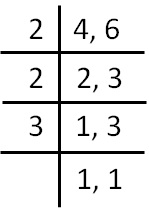LCM of 4 & 6 = 2 × 2 × 3

= 12

## Find LCM of 12 & 16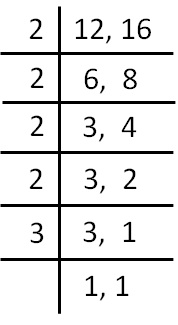LCM of 12 & 16 = 2 × 2 × 2 × 2 × 3

= 4 × 4 × 3

= 48

## Find LCM of 20, 28, 36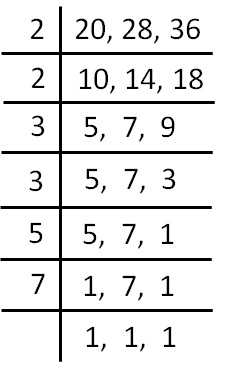LCM of 20, 28, 36 = 2 × 2 × 3 × 3 × 5 × 7

= 4 × 9 × 35

= 1260

## Find LCM of 13 & 23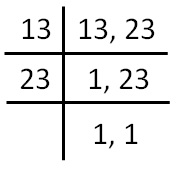LCM of 13 & 23 = 13 × 23

= 299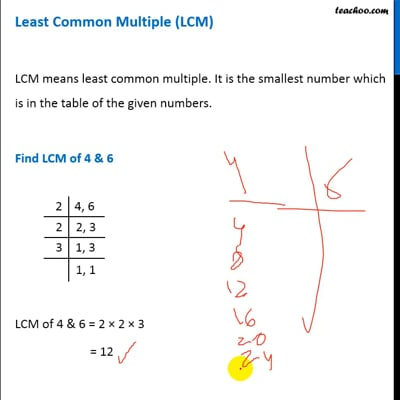This video is only available for Teachoo black users